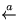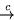Contents

3.3 Driving with Neighborhood

Consider the function fa which looks for the first 'a' in a string and returns T if it is found; otherwise it returns F:

 = T = = F

Consider the computation of <fa 'kasha'>:

 1 2 3 T

One may notice that there is a part of the argument, namely 'sha', which did not take part in computation. It could be replaced by any expression, and the computation, as well as its final result, would not change a bit. One might guess that this kind of information about computational processes may be of interest for different purposes, such as debugging and testing programs. A variation of driving, driving with neighborhood , provides a general method for representing and extracting such information.

We define a neighborhood as the structure (a)p, where p is a pattern and a is a list of assignments for all variables in p, such that the result of substitution a/p is a ground expression (i.e., one without variables) referred to as the center of the neighborhood. Driving with a neighborhood is a combination of computation and driving. In computation the argument is a ground expression, and it defines the path of computation: which sentence is used at each step. In driving the argument is an arbitrary expression, and we analyze all possible computation paths for it. When driving with a neighborhood we drive its pattern, but consider only one path, namely the one taken by the neighborhood's center. At each step the center is the same as if the initial function call were directly evaluated. The free variables in the pattern p (which may be loosely called neighborhood) represent the part of information which was not, up to the current stage, used in computation.

Consider this driving:

 neighborhood function call contraction 1. ('kasha'y) y y'k'y 2. ('asha'y)'k'y y'a'y 3. ('sha'y)'ka'y T

In the initial neighborhood (column 2) the pattern has the maximal extension: anything, a free variable 'y'; the assignment makes the center 'kasha'. In column 3 is the call to compute the pattern. By driving under the definition of  fa, we find that the contraction in column 4 is necessary in order to take the path the center will take (the second sentence of the definition). Modifying the neighborhood by this contraction, we have the next stage neighborhood in line 2. Proceeding further in this manner, we complete the computation of the initial call - it is T - and get the representation of the argument as a neighborhood with the pattern 'ka'y, which tells us that together with our argument, any argument which matches 'ka'y will lead to the same result of computation.

Sergei Abramov found a way to use neighborhood analysis for program testing [1,2]. This may seem strange, because the neighborhoods give us information about unused parts of arguments, data, not about the program. But Abramov makes a metasystem transition: driving with neighborhood is applied not to program P working on data D, but to program Int which is an interpreter of the language in which P is written and works on the pair (P,D). Now the program becomes data. Fixing some input D - a test for P - we can, by neighborhood analysis, determine what parts of the program were used, and hence tested,in this run, and which parts were not.

On this basis Abramov built an elegant theory of program testing, which uses the following principle: choose each next test so as to check those features of the program which have not yet been tested. Abramov gives a precise mathematical definition to this intuitive principle and provides the necessary theorems and algorithms.

Contents  Next